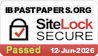# Topic 1: Measurement and uncertainties​

## 1.1 – Measurements in physics

• Fundamental and derived units

Fundamental SI units

Derived units are combinations of fundamental units. Some examples are:

• m/s (Unit for velocity)
• N (kg*m/s^2) (Unit for force)
• J (kg*m^2/s^2) (Unit for energy)
• Scientific notation and metric multipliers

In scientific notation, values are written in the form a*10^n, where a is a number within 1 and 10 and n is any integer. Some examples are:

• The speed of light is 300000000 (m/s). In scientific notation, this is expressed as 3*10^8
• A centimeter (cm) is 1/100 of a meter (m). In scientific notation, one cm is expressed as 1*10^-2 m.

Metric multipliers

• Significant figures

For a certain value, all figures are significant, except:

2. Trailing zeros if this value does not have a decimal point, for example:
• 12300 has 3 significant figures. The two trailing zeros are not significant.
• 012300 has 5 significant figures. The two leading zeros are not significant. The two trailing zeros are significant.

When multiplying or dividing numbers, the number of significant figures of the result value should not exceed the least precise value of the calculation.

The number of significant figures in any answer should be consistent with the number of significant figures of the given data in the question.

### FYI

• In multiplication/division, give the answer to the lowest significant figure (S.F.).
• In addition/subtraction, give the answer to the lowest decimal place (D.P.).
• Orders of magnitude

Orders of magnitude are given in powers of 10, likewise those given in the scientific notation section previously.

Orders of magnitude are used to compare the size of physical data.

• Estimation

Estimations are usually made to the nearest power of 10. Some examples are given in the tables in the orders of magnitude section.

## 1.2 – Uncertainties and errors

• Random and systematic errors

• Absolute, fractional and percentage uncertainties

Physical measurements are sometimes expressed in the form x±Δx. For example, 10±1 would mean a range from 9 to 11 for the measurement.

Calculating with uncertainties

• Error bars

Error bars are bars on graphs which indicate uncertainties. They can be horizontal or vertical with the total length of two absolute uncertainties.

• Uncertainty of gradient and intercepts

Line of best fit: The straight line drawn on a graph so that the average distance between the data points and the line is minimized.

Maximum/Minimum line: The two lines with maximum possible slope and minimum possible slope given that they both pass through all the error bars.

The uncertainty in the intercepts of a straight line graph: The difference between the intercepts of the line of best fit and the maximum/minimum line.

The uncertainty in the gradient: The difference between the gradients of the line of best fit and the maximum/minimum line.

## 1.3 – Vectors and scalars

• Vector and scalar quantities
• Combination and resolution of vectors

Vector addition and subtraction can be done by the parallelogram method or the head to tail method. Vectors that form a closed polygon (cycle) add up to zero.

When resolving vectors in two directions, vectors can be resolved into a pair of perpendicular components.

### FYI

The relationship between two sets of data can be determined graphically.

Topic 1: Measurement and uncertainties​